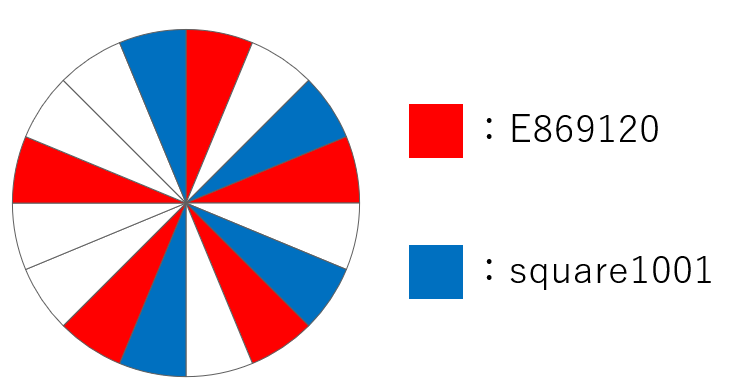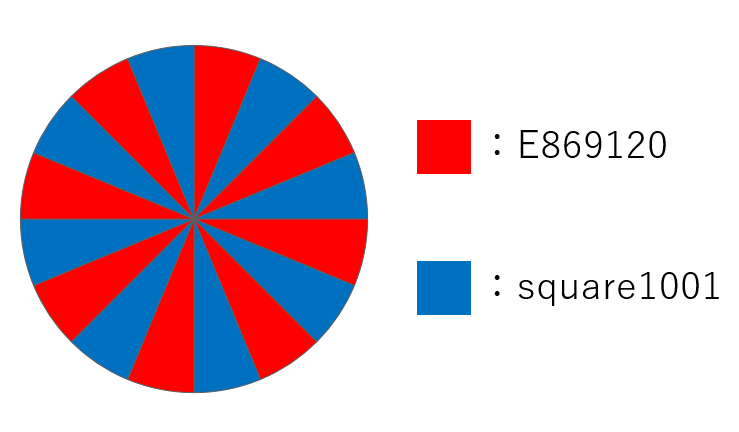# Home

Score: $100$ points

### Problem Statement

E869120's and square1001's $16$-th birthday is coming soon.
Takahashi from AtCoder Kingdom gave them a round cake cut into $16$ equal fan-shaped pieces.

E869120 and square1001 were just about to eat $A$ and $B$ of those pieces, respectively,
when they found a note attached to the cake saying that "the same person should not take two adjacent pieces of cake".

Can both of them obey the instruction in the note and take desired numbers of pieces of cake?

### Constraints

• $A$ and $B$ are integers between $1$ and $16$ (inclusive).
• $A+B$ is at most $16$.

### Input

Input is given from Standard Input in the following format:

$A$ $B$


### Output

If both E869120 and square1001 can obey the instruction in the note and take desired numbers of pieces of cake, print Yay!; otherwise, print :(.

### Sample Input 1

5 4


### Sample Output 1

Yay!


Both of them can take desired number of pieces as follows:### Sample Input 2

8 8


### Sample Output 2

Yay!


Both of them can take desired number of pieces as follows:### Sample Input 3

11 4


### Sample Output 3

:(


In this case, there is no way for them to take desired number of pieces, unfortunately.